### Why does the logdens command improve classification?

Note that this question is not so relevant anymore for PRTools4.2.0 and later as the logdens routine is now always automatically called when applicable in case classc is applied to the classifier (i.e. if posteriors are used instead of densities). Not all help files are updated yet.

Classifiers following the Bayes classification rule may profit from using log-densities instead of densities if they are based on normal distributions. This improvement is not fundamental It is just a computational trick that overcomes some limitations of the finite word length of computers. Here is a short explanation.

The multi-class  Bayes classifier  between classes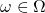can be written as (see the glossary)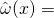argmax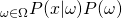The result (the class with the largest posterior probability) does not change if in the argument of the argmax function a positive monotonic transformation is included. Let us take the logarithm:argmax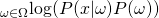For normal distributions with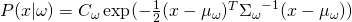this is equivalent toargmax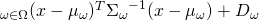as the logarithm cancels the exponent and all constants which are independent of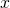can be collected in a single constant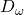.

The above shows that the logarithmic formulation of the Bayes classifier is equivalent to the original one. The numeric implementations, however, may give different results in high-dimensional spaces. PRTools tries to compute proper densities in the procedures based on the Bayes classifier. So +testset*qdc(trainset) shows the densities of the objects in testset estimated from trainset.

In high-dimensional spaces these densities however can become very small. Due to the finite word length the density estimates based on exponents may become identical (at the end even zero) for different classes. Objects are thereby not optimally classified. Avoiding the exponent can be profitable in the tails of the distributions. This also holds for the density estimates based on sums of exponents like in mogc and parzenc. Formally the logarithm does not cancel the exponents in a sum of exponents. In practice however the contribution of a single exponent dominates in the tail of the total distribution. All others can thereby be neglected.

In PRTools density based classifiers can be called in two modes: without or with classc. In the first case proper densities are estimated and using the logarithm would spoil this. In the second case classc takes care that posteriors are computed instead of densities. The computation of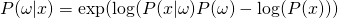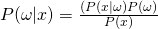is included in the call to classc for the recent versions of PRTools. Users don’t have to call logdens themselves if they call classc. The example prex_logdens shows the difference between classifiers without and with logdens that are not based on classc.